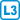## Non-standard fair dice

Construct two dice, each has 6 faces and each face has at lest one pip, with the following property: For any $$k$$, the probability that $$k$$ pips falls together after a roll of these two dice is the same as for a roll of a pair the standard (honest) dice.

These two dice shall not be the standard ones, but the same number of pips may occur on more faces of the same dice.

• #### Hint

First, describe the sequence of probabilities by a suitable generating function. Then split this function into two to match the non-standard dice you are looking for.

• #### Solution

When throwing two honest standard dice, the probability that the sum of $$k$$ falls is equal to the coefficient by $$x^k$$ in the following polynomial: $P(x) = \frac{1}{36}(x + x^2 + x^3 + x^4 + x^5 + x^6)^2.$ This polynomial fould be adjusted as $\begin{equation*} \begin{split} P(x) &= \frac{1}{36}x^2(1 + x + x^2 + x^3 + x^4 + x^5)(1 + x + x^2 + x^3 + x^4 + x^5) \cr &= \frac1{36}x^2(1+x+x^2)(1 + x^3)(1+x)(1 + x^2 + x^4). \end{split} \end{equation*}$ Observe that in the first factor we have extracted $$1+x+x^2$$, while in the second we have extracted $$1+x$$ (both are possible). Now we rearrange the terms and get: $\begin{equation*} \begin{split} P(x) &= \frac1{36}x(1+x+x^2)(1 + x) \cdot x(1+x^3)(1 + x^2 + x^4) \cr &= \frac1{36}(x + 2x^2 + 2x^3 + x^4) \cdot (x + x^3 + x^4 + x^5 + x^6 + x^8). \end{split} \end{equation*}$ From this we see that if we create two fair dice, the first die with 1, 2, 2, 3, 3, and 4 pips and the second die with 1, 3, 4, 5, 6, and 8 pips, then the probability that the roll of these two dice yields $$k$$ pips is equal to the coefficient by $$x^k$$ in the polynomial $$P(x)$$. This yields the required dice.